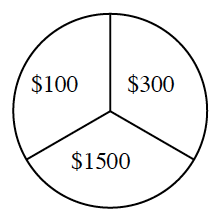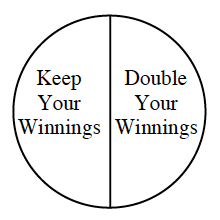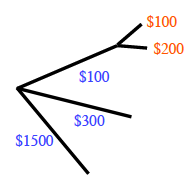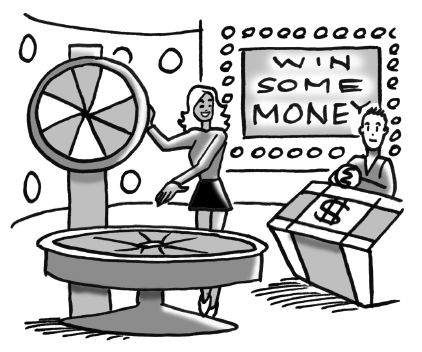### Home > CCG > Chapter 3 > Lesson 3.2.3 > Problem3-79

3-79.

Congratulations! You are going to be a contestant on a new game show with a chance to win some money. You will spin the two spinners shown below to see how much money you will win.1. Make a tree diagram of all the possible outcomes of spinning the two spinners. At the ends of the branches, on the far right, write the amount you would win for each combination of spins.2. Are each of the outcomes in the sample space equally likely?

Do any outcomes appear more than once? In each spinner, is any result more likely than the other(s)?

3. What is the probability that you will take home $\200$? What is the probability that you will take home more than $\500$?

$\frac{1}{6},\frac{3}{6}$

4. What is the probability that you will double your winnings? Does the probability that you will double your winnings depend on the result of the first spinner?

Look at the second spinner by itself.

5. What if the amounts on the first spinner were $\100$, $\200$, and $\1500$? What is the probability that you would take home $\200$? Justify your conclusion.

Draw a second tree diagram to avoid becoming confused.Use the eTool below to plot points and draw the line.
Click the link at right for the version of the eTool.  3-79 HW eTool